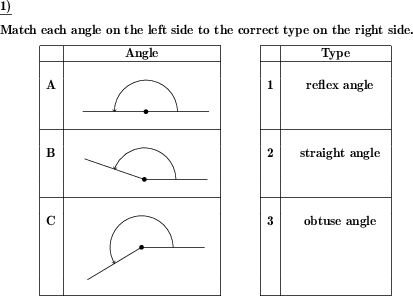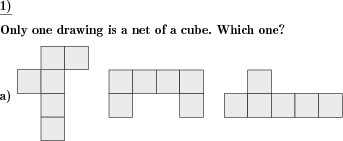Custom math worksheets at your fingertipsDetails for problem "Triangle label parts"

Quickname: 3233

Junior High School, Middle School, High School.

Summary

A fully or partly unlabeled triangle is given. The vertices, sides or angles have to be labeled correctly.

ExampleDescription

Excercise a basic concept of geometry, label parts of a given triangle. A triangle is given. Parts of the triangle have to be labeled.

Parts that can be asked for are

• vertices
• sides
• angles

Mastery of this concept is a must by providing this worksheet to the independent learners and then in small groups to brainstorm vertices, sides, and angles of any given triangle. A variety of problems can be generated to use as a test paper for grade 7 and grade 8. Building a strong concept of this worksheet will grasp this array of targets.

1. Identify parts of a triangle.
2. Differentiate sides, vertices, and angles of a given triangle.
3. Explore the notations/ symbols used to label all three parts.
4. Develop a base for more complex geometry concepts.

Adjust the level of complexity by generating this worksheet according to the ability level of learners. The number of problems can range from 1 to 10. For every type of part, it can be chosen whether

• all of these are given, e.g. for reference when the labels of other parts are left blank
• none are given but they are asked for
• none are given and they are not asked for
• exactly of these is given (e.g. one side's label, one angle's label etc)

The solution sheet will show the triangle with all parts labeled.

Download free printable worksheets for this math problem here. The worksheet contains the problems only, the solution sheet includes the answers. Just click on the respective link.

•Worksheet 1Solution sheet with answers
•Worksheet 2Solution sheet with answers
•Worksheet 3Solution sheet with answers

If you can not see the solution sheets for download, they may be filtered out by an ad blocker that you may have installed. If this is the case, please allow ads for this page and reload the page. The solution sheets will then reappear.

• Do these sample worksheets do not really fit?
• Do you need more math worksheets, with a different level of difficulty?
• Would you like to combine different problems on a worksheet and adjust them to your needs?
• As a teacher, you can put together your own worksheets using the automatically generated math problems provided.
With a free initial credit, you can start creating your own math worksheets in a few minutes.

You can try it for free! Register here, to create custom worksheets now!

Customization options for this problem

Parameter
Possible values
Number of problems
1, 2, 3, 4
Sides
do not ask, all given, none given, one given
Angles
do not ask, all given, none given, one given
Vertices
do not ask, all given, none given, one given

dw-Math worksheet templates that do contain this problem

Name
Title
Description
Label the basic parts of a triangle
This work package is about the basic parts: Vertices, angles and sides.

Other types of problems that appear on worksheets with this problem:

Relevance
Name
Description
Quickname
Example
****
Angle types
For a given angle, the correct type has to be marked.****
Match angles to angle types
Given angles have to be matched to given types****
Identify cube nets in a series of cube-like nets
A series of drawings is shown. Which ones represent cube nets?****
Triangle draw incircle
The incircle and angle bisectors have to be drawn for a given triangle.Deutsche Version dieser Aufgabe
These informational pages with samples describe math problems that can be combined on custom math worksheets with solutions for home and K-12 school use.
Deutsche Seiten
×